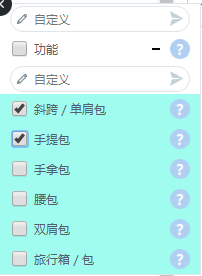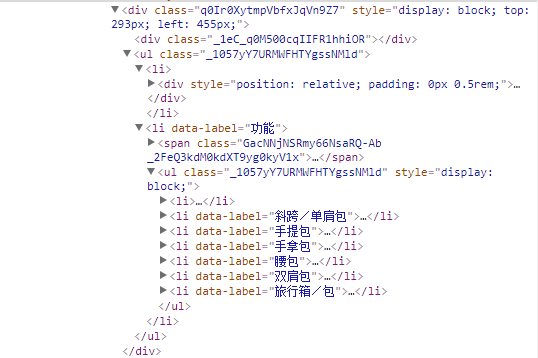javascrpit复选框怎么用键盘来代替鼠标的点击事件 50C9个回答

html代码如下：

jquery代码如下：
\$('html').keydown(function(event) {
if (event.which >= 49 && event.which <= 57) {
\$num = event.which - 49;
\$('input[name=check]:eq(' + \$num + ')').prop("checked")?\$('input[name=check]:eq(' + \$num + ')').prop("checked",false):\$('input[name=check]:eq(' + \$num + ')').prop("checked",true);
}
})
});Forsaken12345 这不是我的网站，怎么在chrome的控制台使用

html代码

<input type="checkbox" name="check">
<input type="checkbox" name="check">
<input type="checkbox" name="check">
<input type="checkbox" name="check">
<input type="checkbox" name="check">
<input type="checkbox" name="check">
<input type="checkbox" name="check">

keydown() 键盘按下触发事件

jquery代码如下：
\$('html').keydown(function(event) {
if (event.which >= 49 && event.which <= 57) {
\$num = event.which - 49;
\$('input[name=check]:eq(' + \$num + ')').prop("checked")?\$('input[name=check]:eq(' + \$num + ')').prop("checked",false):\$('input[name=check]:eq(' + \$num + ')').prop("checked",true);
}
})
});